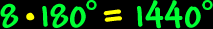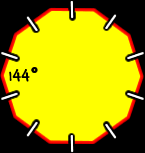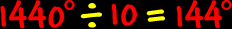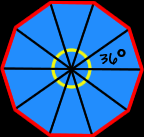# Polygons - Decagons

Properties of decagons, interior angles of decagons

Polygons:  Properties of DecagonsSum of the Interior Angles of a Decagon:
 This image shows the process for a HEXAGON:Using the same methods as for hexagons to the right (I'll let you do the pictures)... To find the sum of the interior angles of a decagon, divide it up into triangles... There are eight triangles...  Because the sum of the angles of each triangle is 180 degrees...  We getSo, the sum of the interior angles of a decagon is 1440 degrees.Regular Decagons:
The properties of regular decagons:All sides are the same length (congruent) and all interior angles are the same size (congruent).

To find the measure of the angles, we know that the sum of all the angles is 1440 degrees (from above)...  And there are ten angles...So, the measure of the angle of a regular decagon is 144 degrees.The measure of the central angles of a regular decagon:To find the measure of the central angle of a regular decagon, make a circle in the middle...  A circle is 360 degrees around...  Divide that by ten angles...So, the measure of the central angle of a regular decagon is 36 degrees.